# EuroEconomica, Vol 33, No 2 (2014)EuroEconomica

Issue 2(33)/2014 ISSN: 1582-8859

The localization problem

Cătălin Angelo IOAN1, Gina IOAN2

1Danubius University of Galati, Department of Economics, catalin_angelo_ioan@univ-danubius.ro

2Danubius University of Galati, Department of Economics, ginaioan@univ-danubius.ro

Abstract. This paper solve completely the problem of determining a point that realizes the minimum weighted sum of the distances to an arbitrary number of points. There are treated the two cases corresponding to existing roads - using graph theory and where roads will built later - by analytical methods. The latter problem is solved, in principle, for n points and practical for three points.

Keywords: location, optimal

1 Introduction

The Localization Problem is of great practical importance. For a proper understanding of it we will, first, give an example.

Consider a firm that produces a good in a quantity Q wishing its distribution to beneficiaries in the quantities Q1,...,Qn. Distribution activity involves the use of means of transport (say, for example, trucks), assumed identical as if skills and performance. Distribution to the beneficiary k will require a number tk=trucks ifis integer and tk=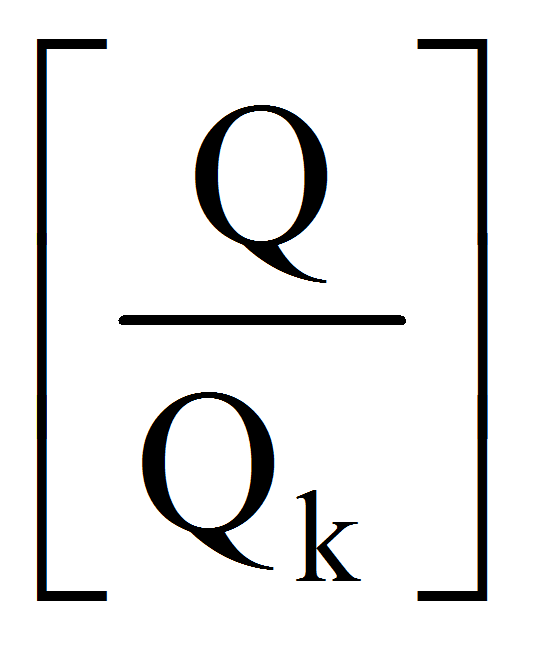+1 trucks otherwise, where [a] is ​​the integer part of the number (i.e. the largest integer less than or equal to a).

The problem lies in determining the location of company headquarters so that total transport costs from the company to the beneficiaries to be minimal. Considering the points Mk of location of beneficiaries and M – the company site, h – the fuel for 1 km with load and s – without load, the total cost of transport (considering that after delivering, the trucks will return to the headquarter) will be: E=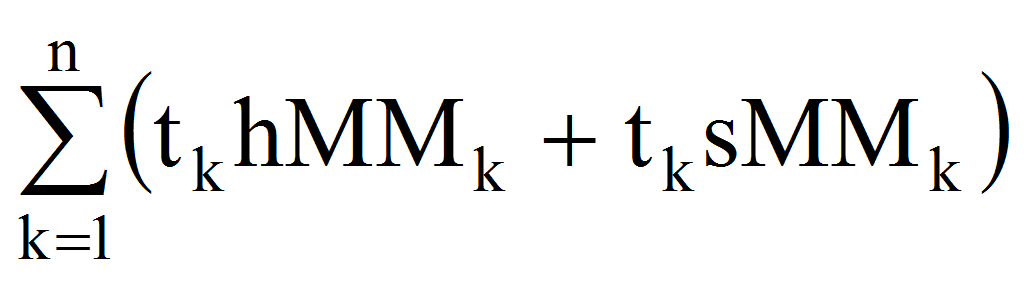. Noting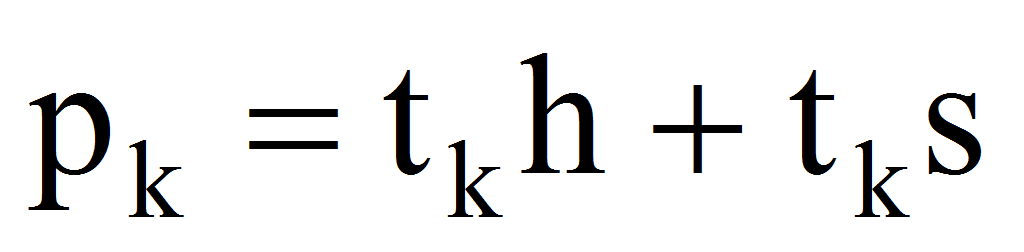, the problem lies in the determination of M for which E=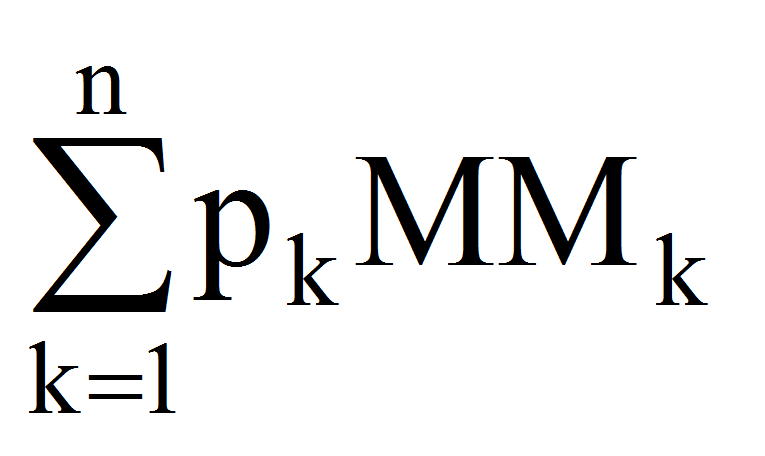=minimal.

The problem presented can be solved in many cases. If there is a network of roads then it returns to the determination of the minimum length of the road in a graph, and if there is not exist, as if in the problem of the arrangement of a base at a site of production, the paths being replaced by the conveyor and being constructed after solving the problem, it is reduced to a purely geometric.

At the end of this introduction, note that the problem is not new, being made ​​in the mid-century XVII by Pierre de Fermat in a letter to Evangelista Torricelli challenging him to determine a point for which the sum of distances to the vertices of a triangle be minimal. The problem was solved geometrically leading to the so-called Fermat-Torricelli point. Subsequently, the problem was generalized in the sense above, proposing that the determination of the weighted average of the distances from the vertices of a triangle to be minimal. Solving the latter problem has benefited also from a purely geometric approach ().

In the following, we will solve the issue presented in several aspects. First, we will completely solve the problem using graph theory, then we will attack the Euclidean appearance that does not imply predetermined roads. In the latter case, we obtain a series of results for both the n points problem and we will give the complete solution for 3 points but by purely analytical methods.

2 The solution of the problem using graph theory

Let the fixed nodes Mk, k=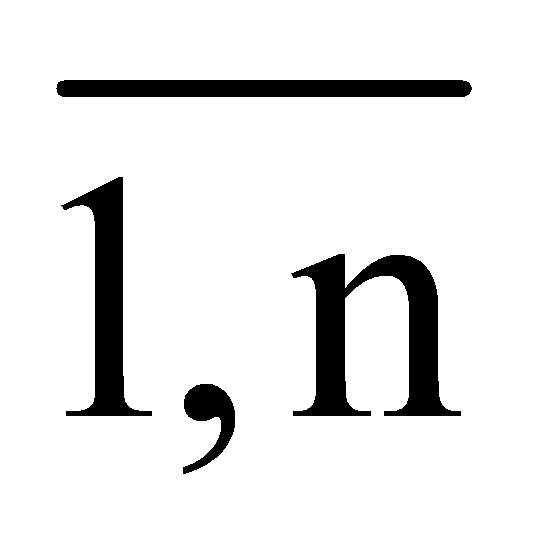, n2 and the nides of potential location Ns, s=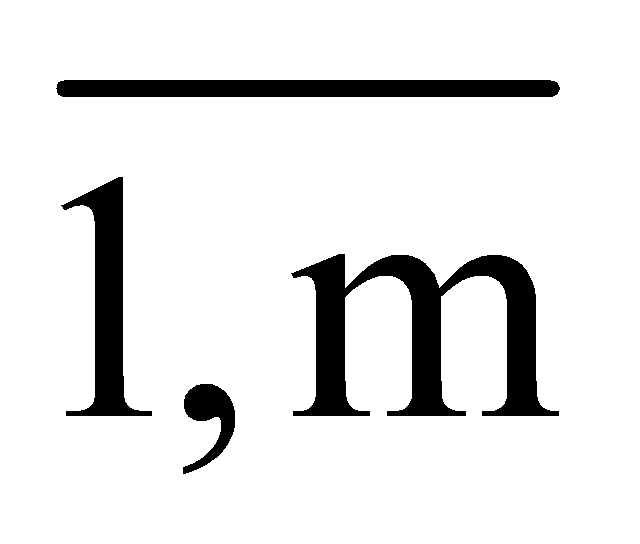, m1, conveniently chosen in a region surrounding the points Mk so that the problem does not impossible charge with potential points (of course in the minimal sense). To solve the problem we will apply Bellman-Kalaba algorithm ().

We will build therefore, for each node Ns, the effective distances matrix Ds,1=(dij), dij=d(Mi,Mj), i,j=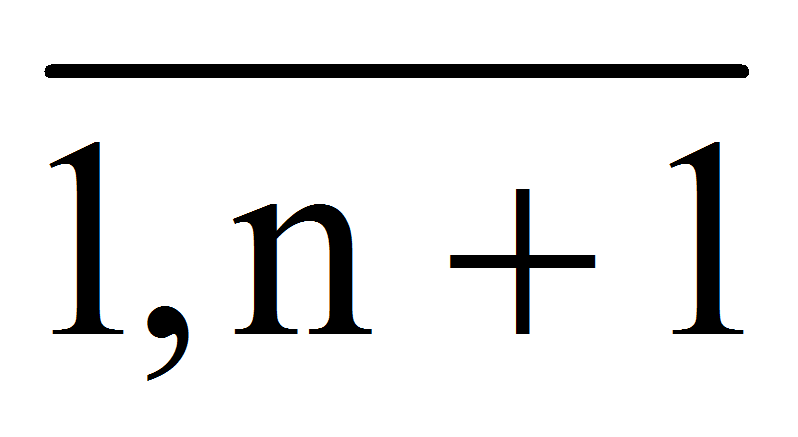where Mn+1=Ns, and d(Mi,Mj) is the length arc connecting Mi to Mj if exists, d(Mi,Mj)=¥ if between Mi and Mj is no arc and d(Mi,Mi)=0. Note now- the minimum length of roads from Mi to Mn+1 consisting of a single arc. Obviously, they are in the column "n +1" of the matrix Ds,1. If we note now at the step p:- the minimum length of roads from Mi to Mn+1 consisting of at most p arcs, we have: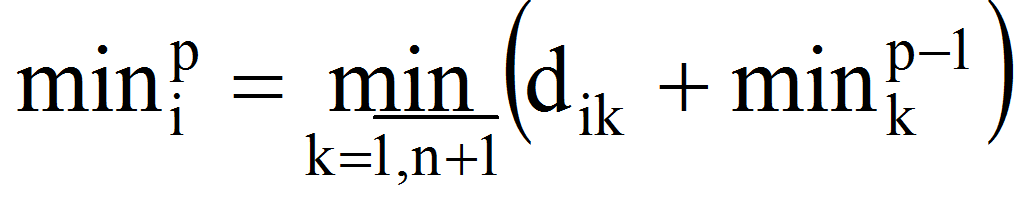. It is clear that unless there is a path between Mi and Mn+1 with at most p arcs we get=¥. To do this, we will construct the matrix Ds,p obtained from the addition of each line of the matrix Ds,1 of the vector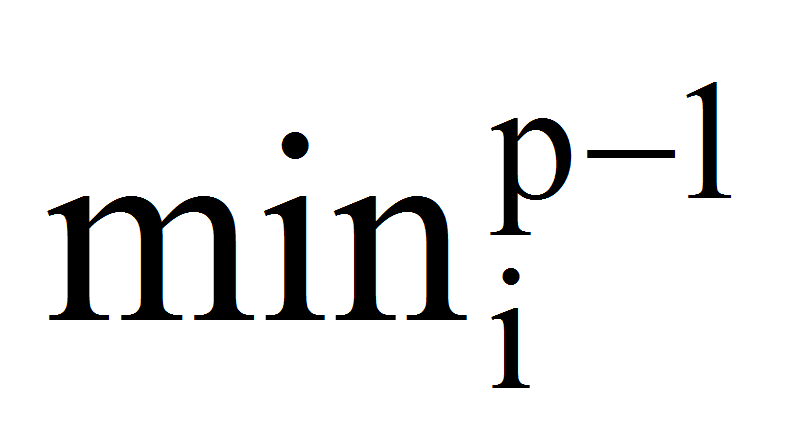. The vectorwill be obtained from the matrix Ds,p by finding the minimum of the elements in the i-th line. The process is continued till we will obtain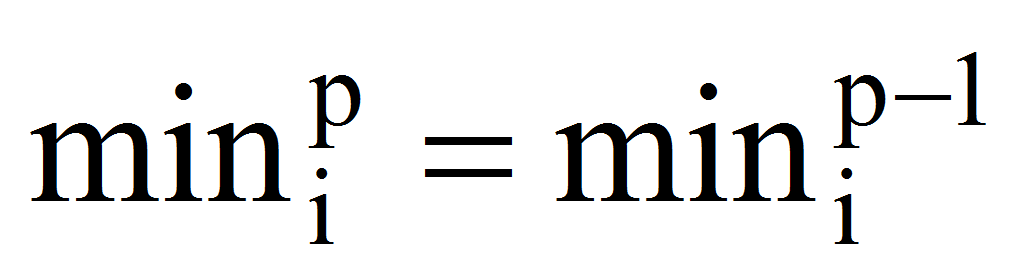, i=. Finally, the vector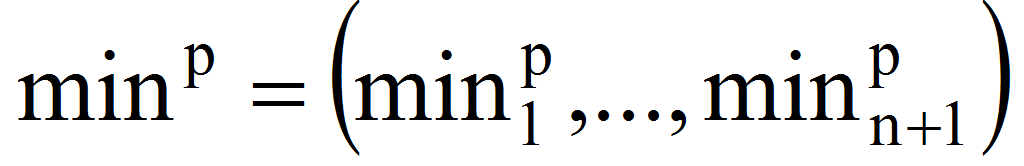will have like components the minimal distances from Ns to each of the points Mk, k=. After this, we will compute Es=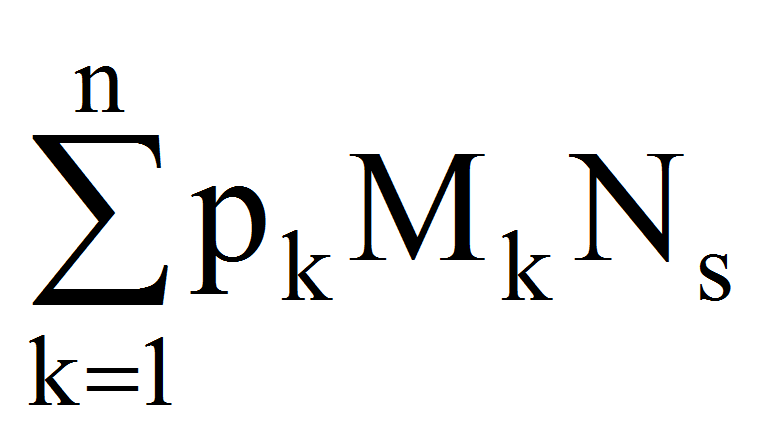=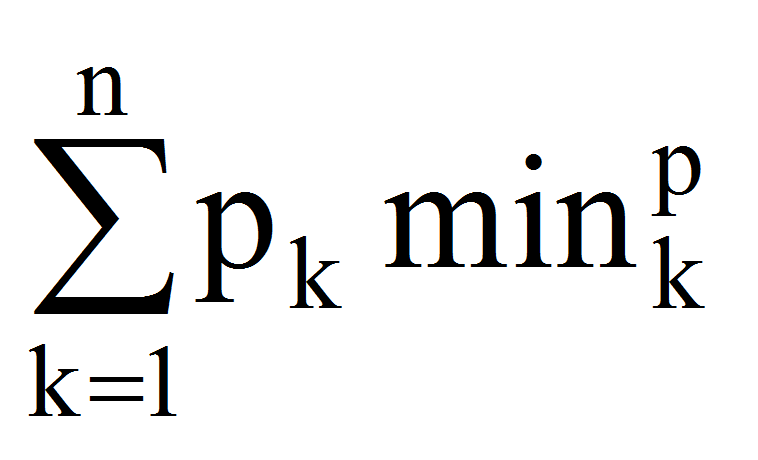. Doing this for all all potential nodes Ns, s=, is determined, finally, that for which is obtained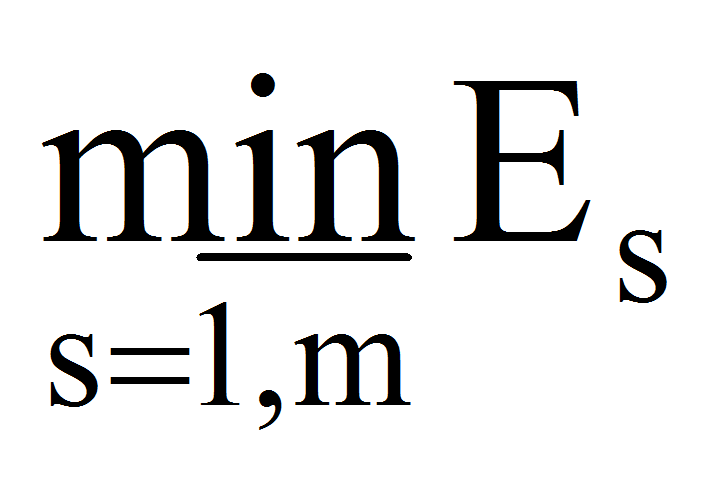.

3 The solution of the general problem

Let the points Mk(xk,yk)R2, k=, pk0, k=. Considering an arbitrary point M(x,y)R2, we formulate the question of determining it such the expression: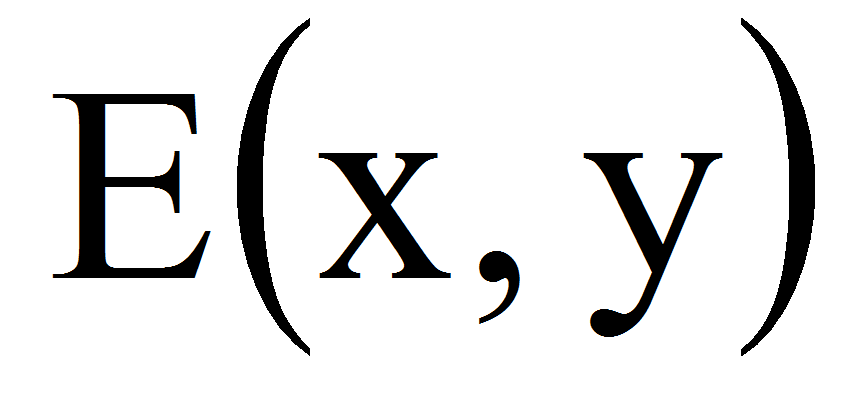=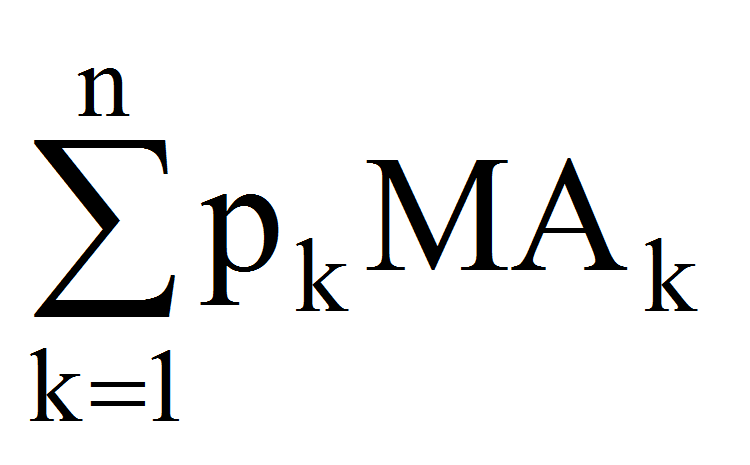=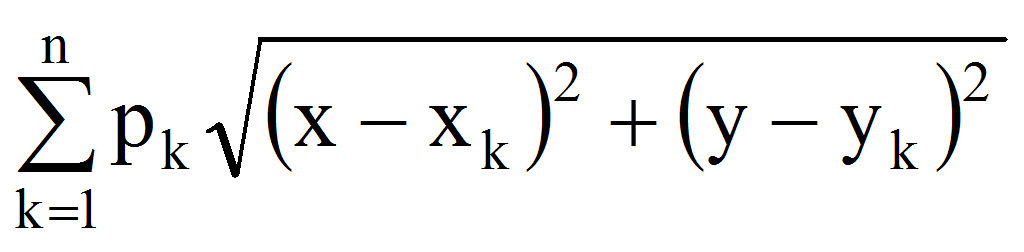(1)

be minimal.

Suppose first that i=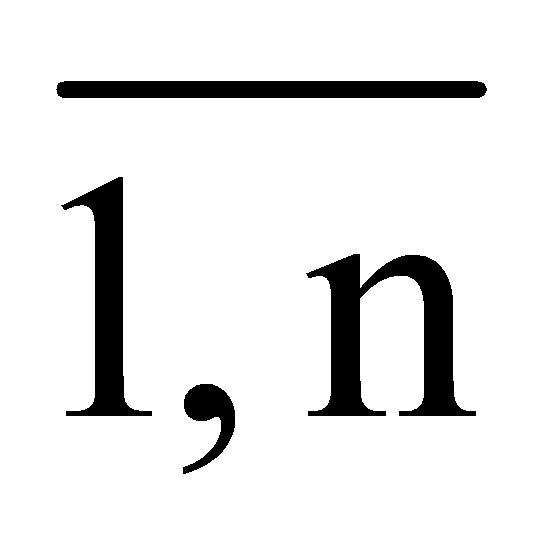such that: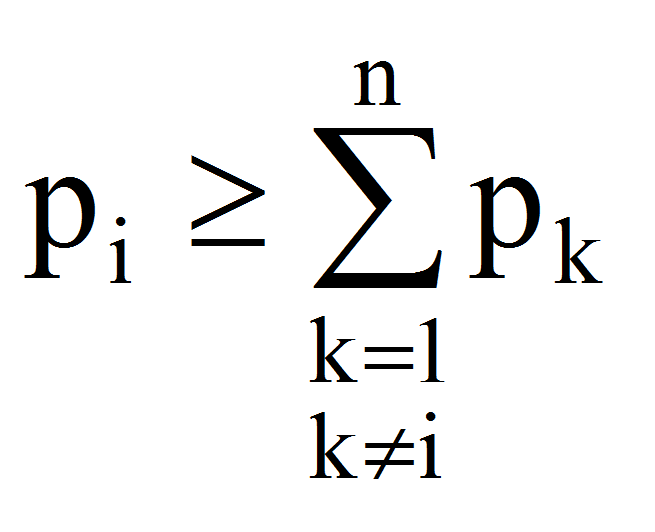. Consider now the expression: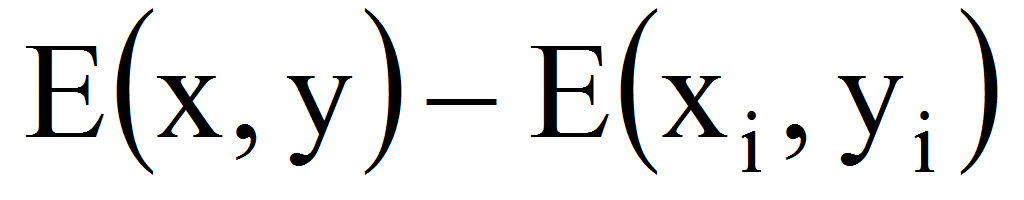=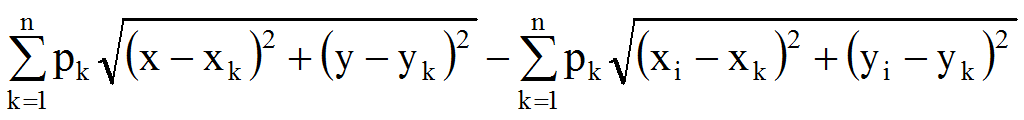=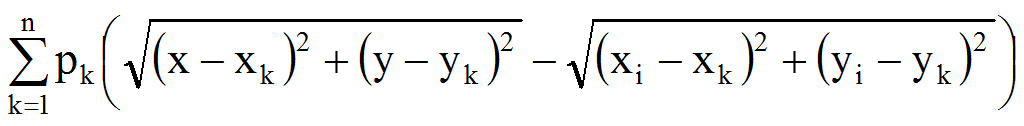(2)

Because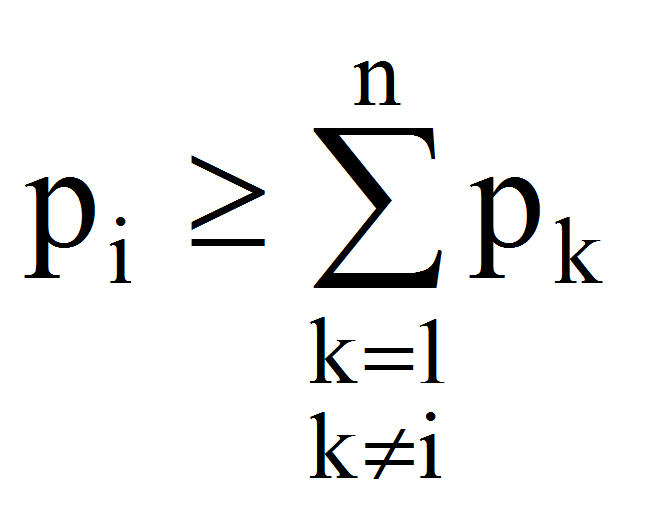let note: u=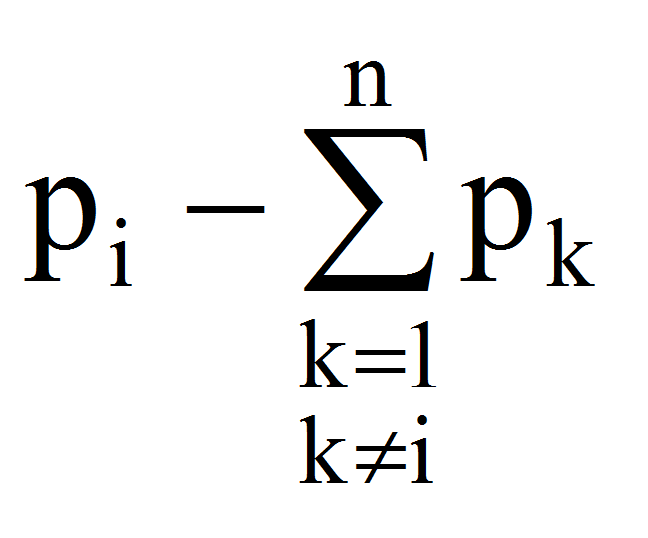0.

We have therefore:=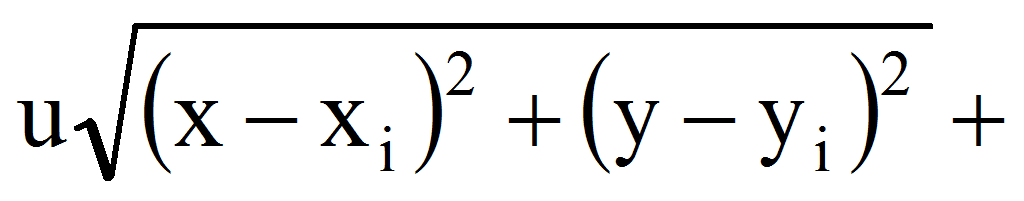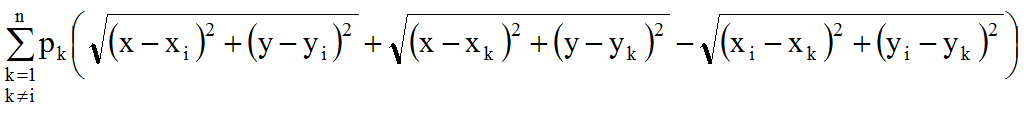(3)

We will prove that: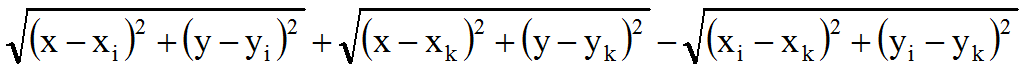0.

Noting: a=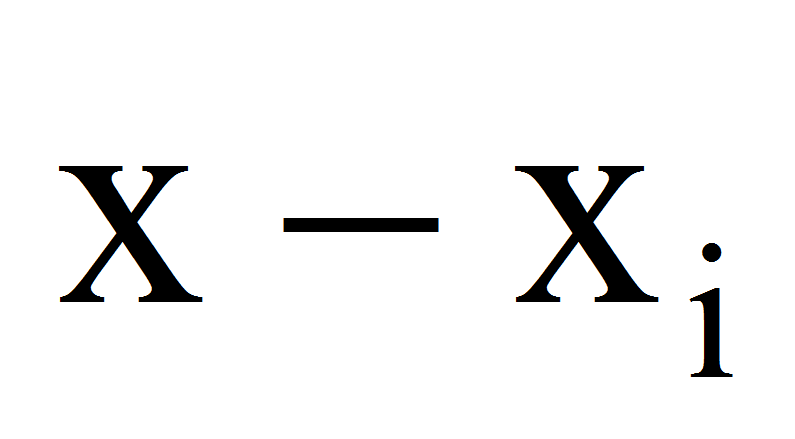, b=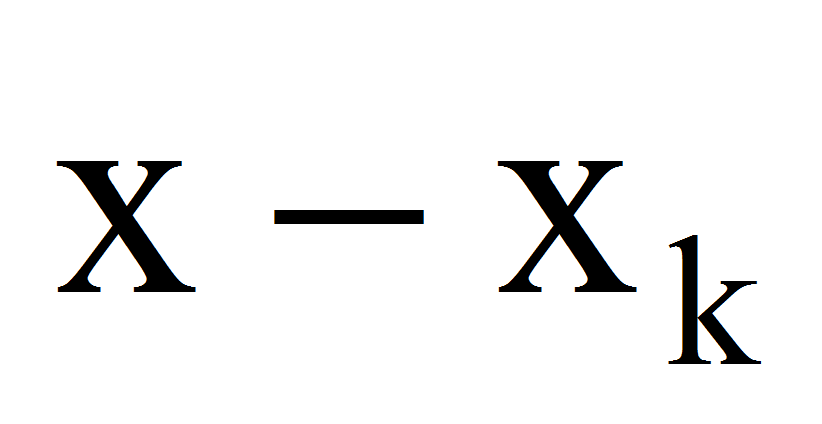, c=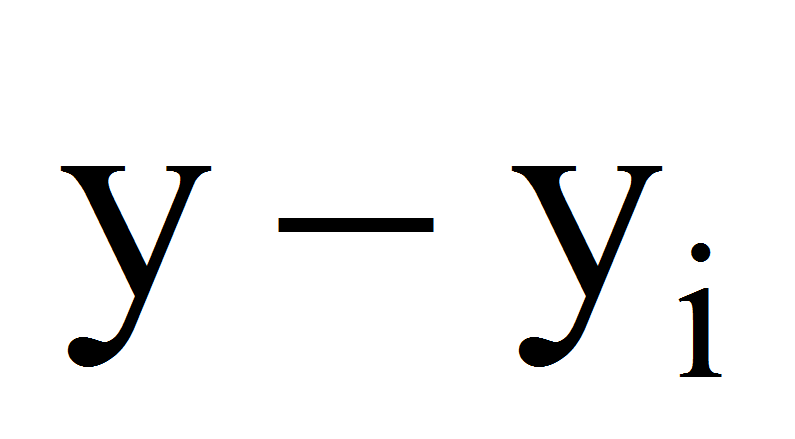, d=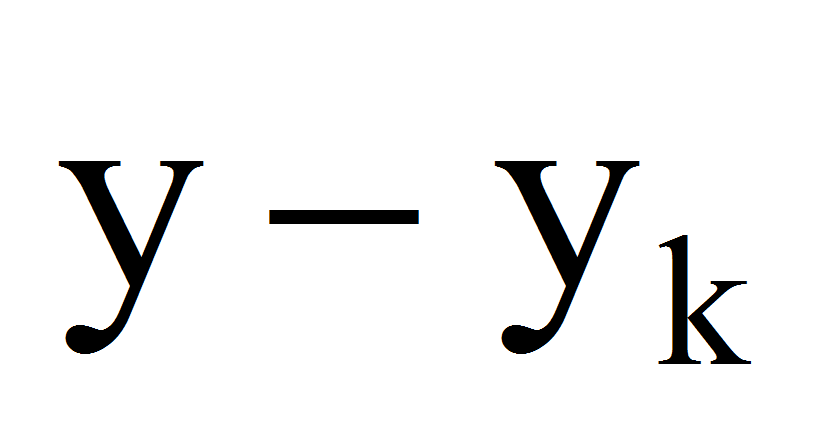the inequality becomes: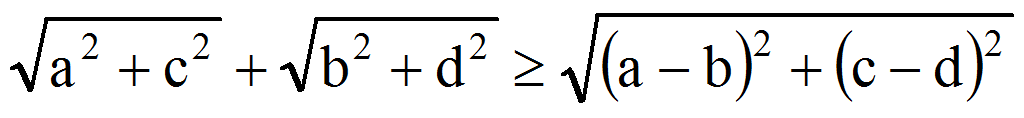and after squaring, this is equivalent to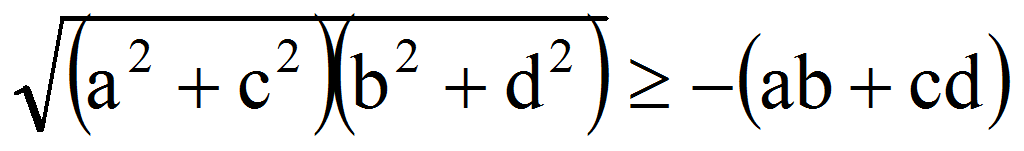. If ab+cd0 then the statement is true, and if ab+cd0 then, after further squaring, it becomes: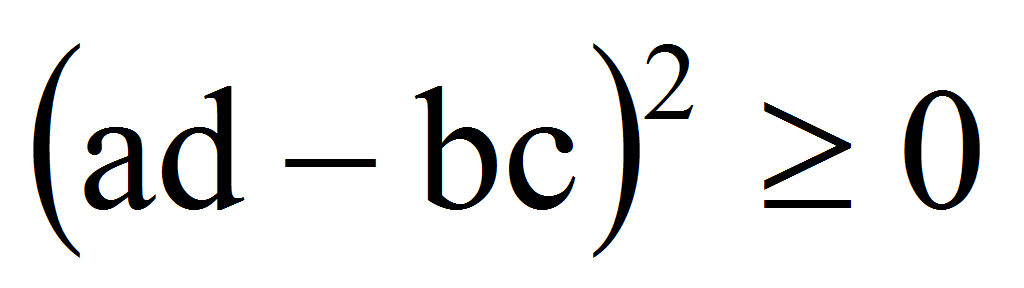- true.

Therefore,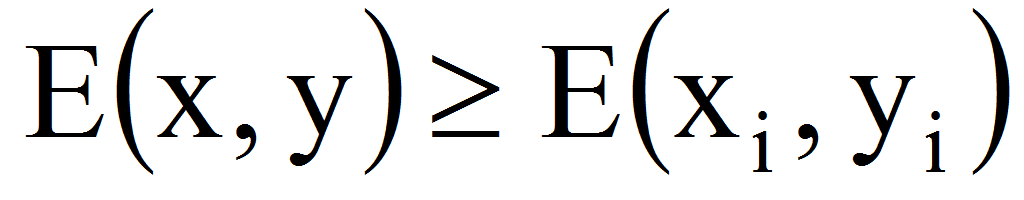(x,y)R2 so ifthen the expression is minimum is reached at the point Mi(xi,yi).

It is obvious that due to the positivity of the quantities pk, k=can not exist at least two indices ij such that,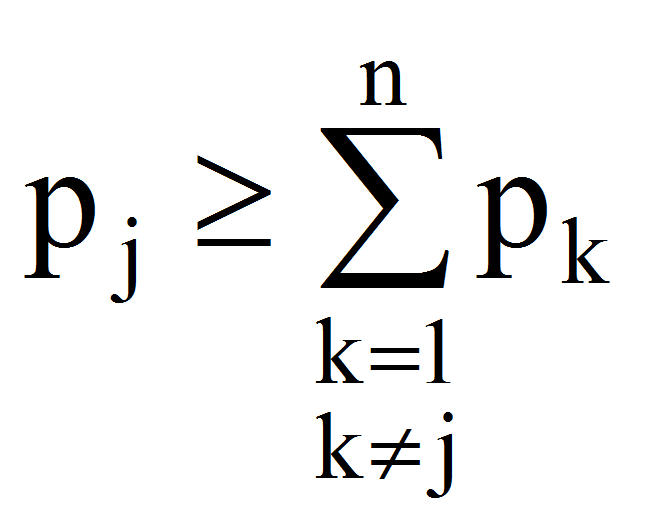.

We therefore consider further that: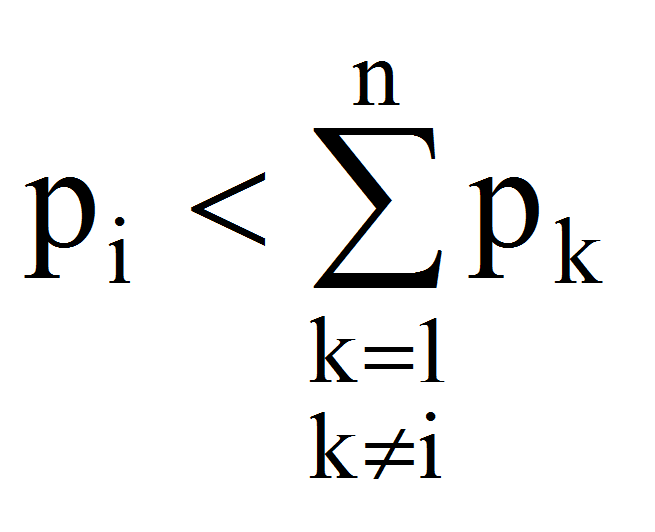, i=.

Compute now, first, the values: Ek=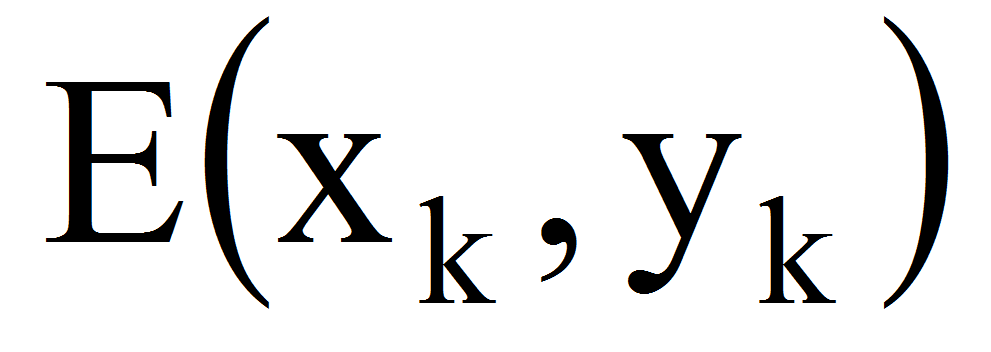and minE=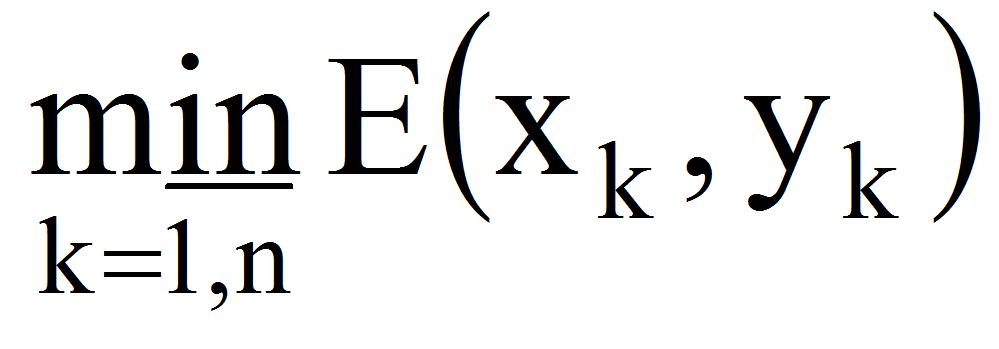and suppose in what follows that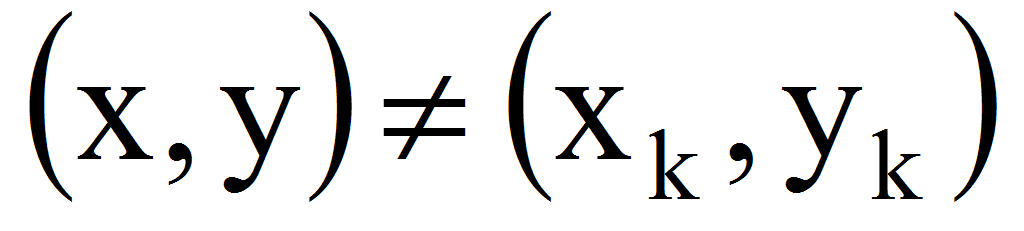, k=. We have now: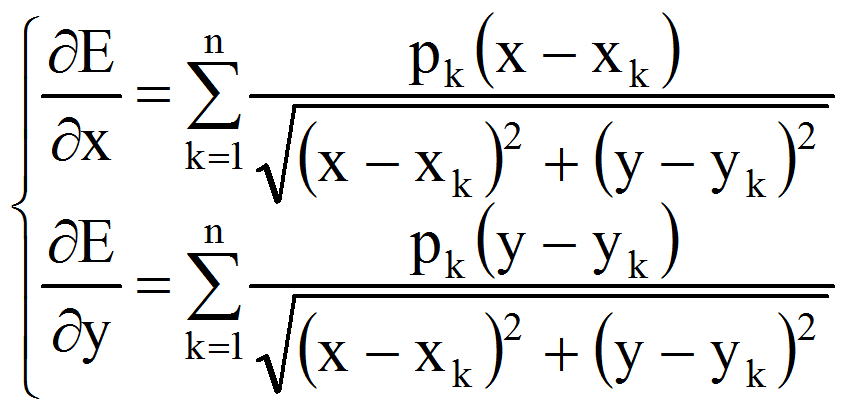(4)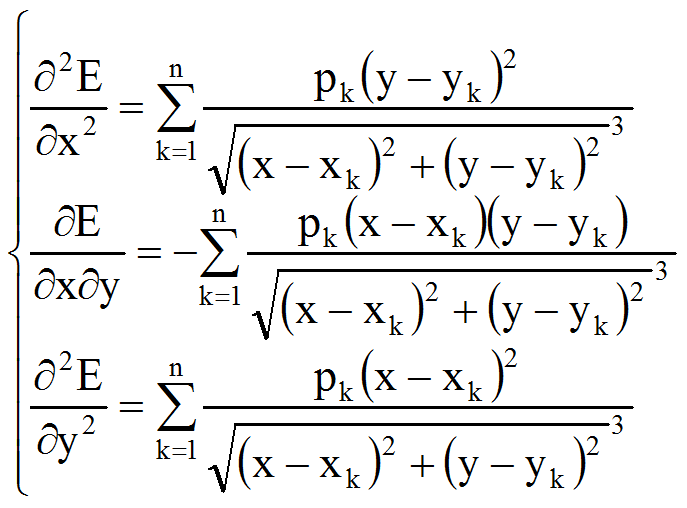(5)

Considering the Hessian matrix: HE=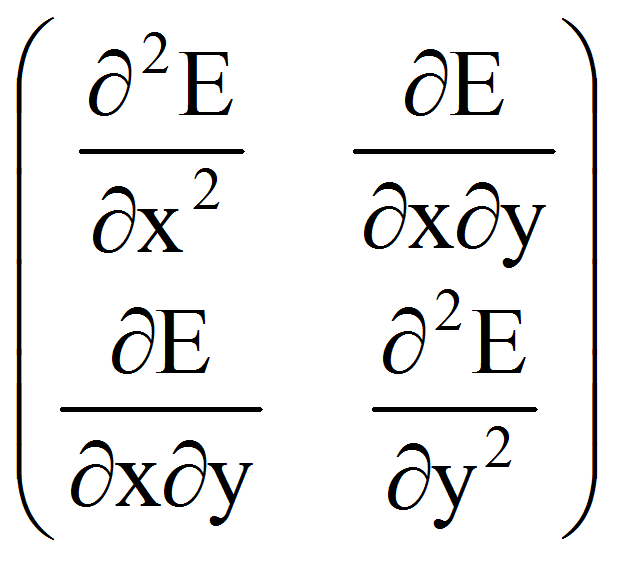, the values of principal diagonal determinants are:

1=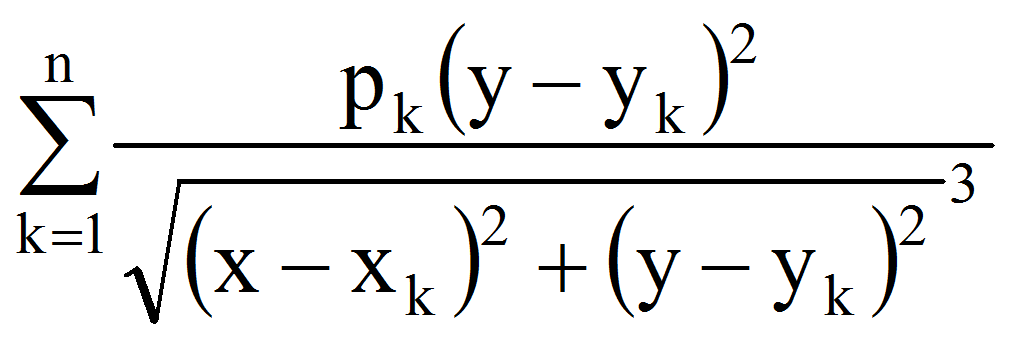0, 2=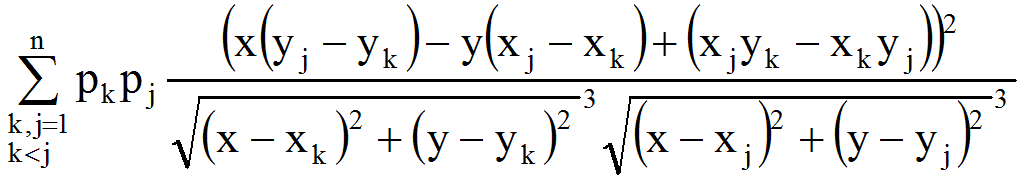0 (6)

so any stationary point will be a local minimum. Furthermore, since the function is strictly convex, it will have at most one global minimum point.

The problem thus reduces to determining the stationary points of E. If none exist, the minimum function E will be minE, and if they are (considering, for example, a point with coordinates (, )) then the minimum will be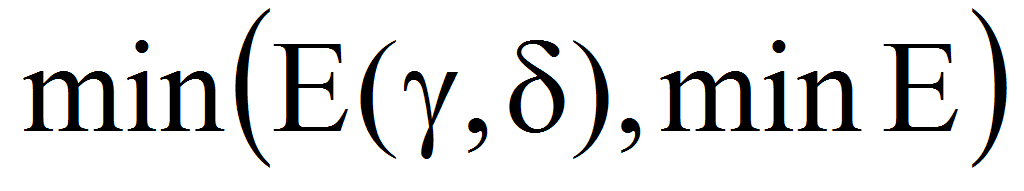.

To determine the stationary points, the solve of the characteristic system is very difficult, in practice requiring computer implementation, but occurring complications relative to the convergence of the algorithms.

We can obtain an approximate solution as follows ():

Let the function f(x,y)=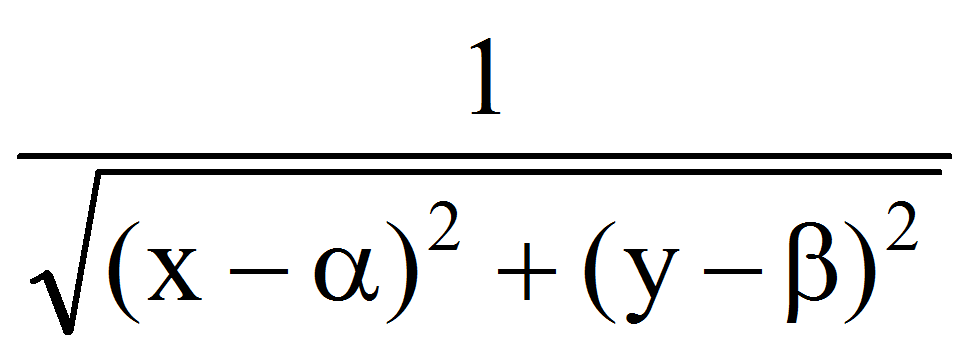. Its development in Mac-Laurin series give:

f(x,y)=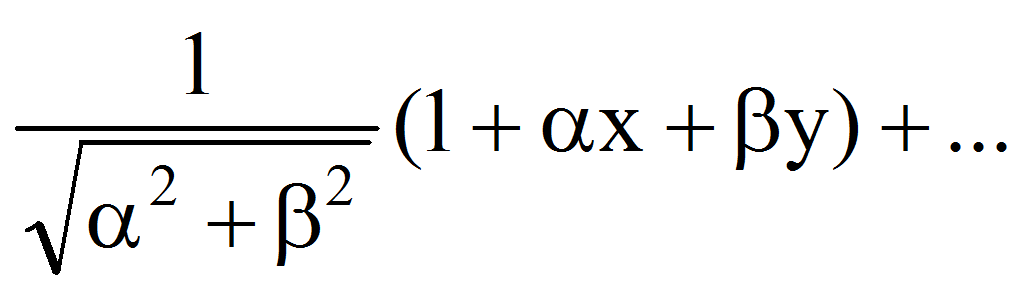Substituting in the characteristic system, we obtain with the notations di=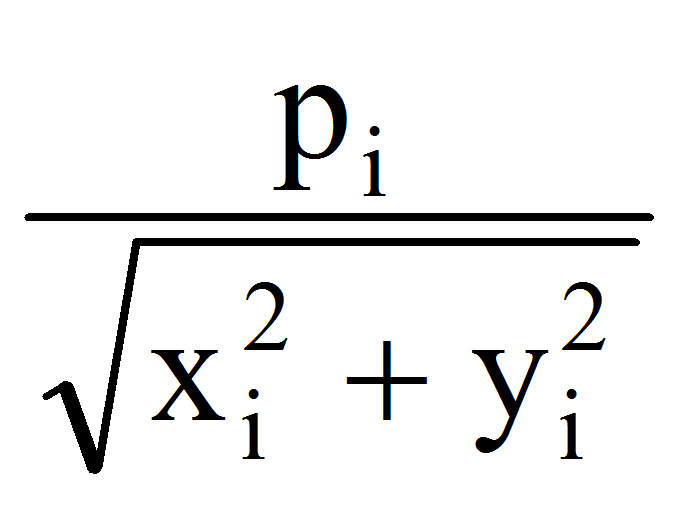, i=: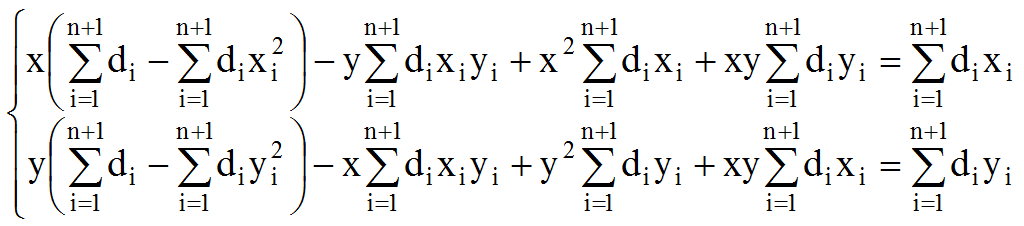(7)

By eliminating y between the two equations we obtain an equation of degree four in x that can provide approximate solutions. Calculating the value of E in these pairs (x,y) and considering the minimum values ​​obtained for one of the pairs (,), we obtain finally:.

The presented method does not claim to provide the exact answer, but it is an approximation of the actual location.

Returning to the original problem, we note now: z=x+iyC and zk=xk+iykC, k=. The determination of stationary points thus reduces to solving the equation: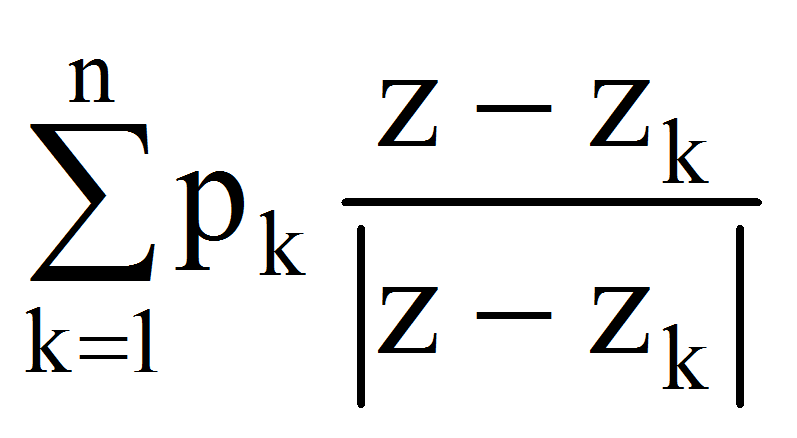=0 (8)

From the fact that, k=follows : zzk, k=therefore z-zk0.

Let now the trigonometric forms of complex numbers: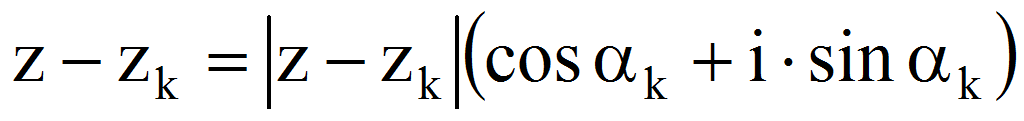,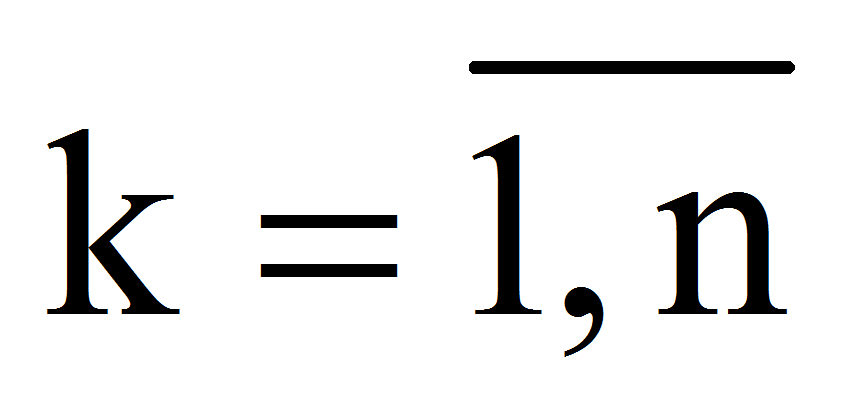.

The equation becomes: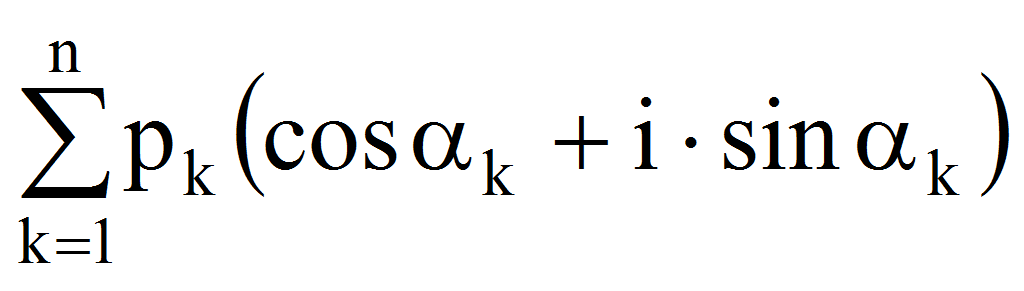=0 (9)

from where: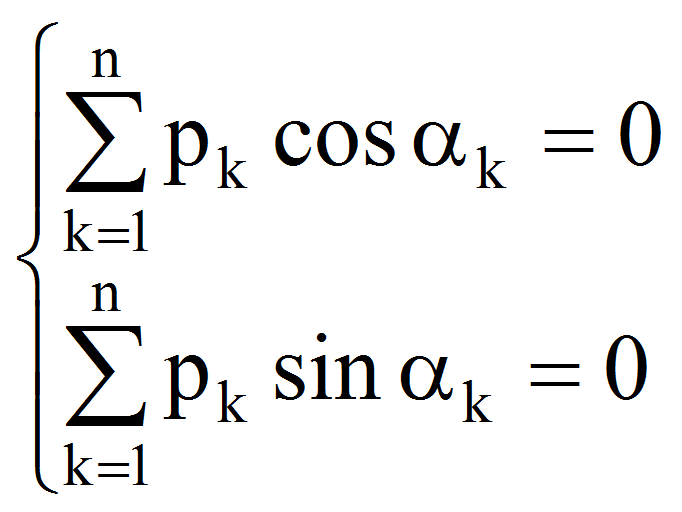(10)

Considering an arbitrary solution of the system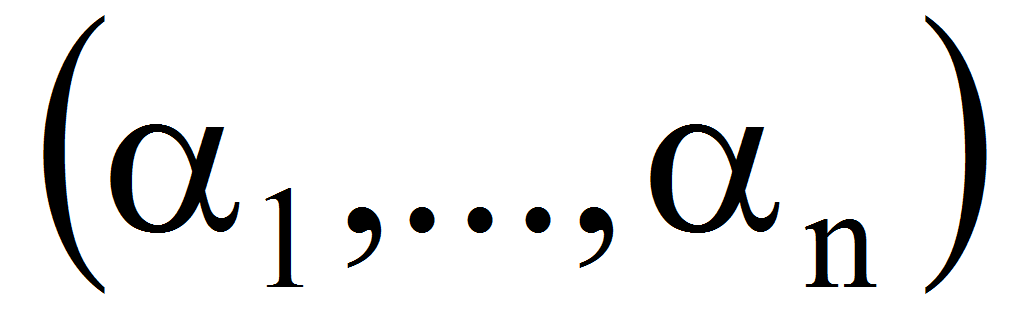,the stationary point will be at the intersection of the lines that pass through zk and with slope tg k, k=.

Consider then, for krs, the straight lines: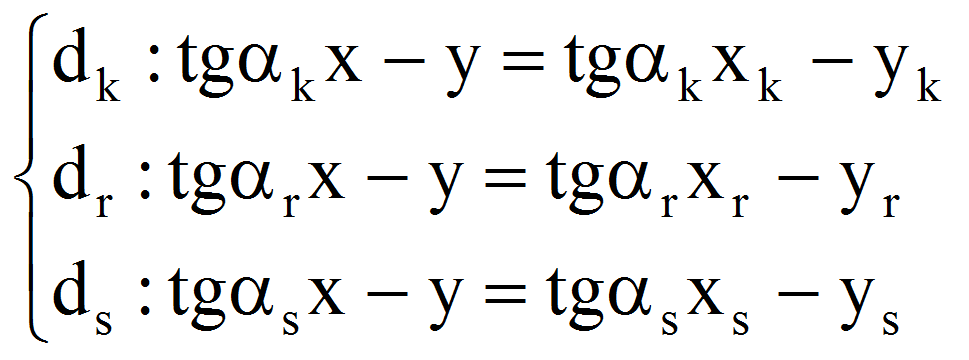(11)

the condition of intersection of all three is: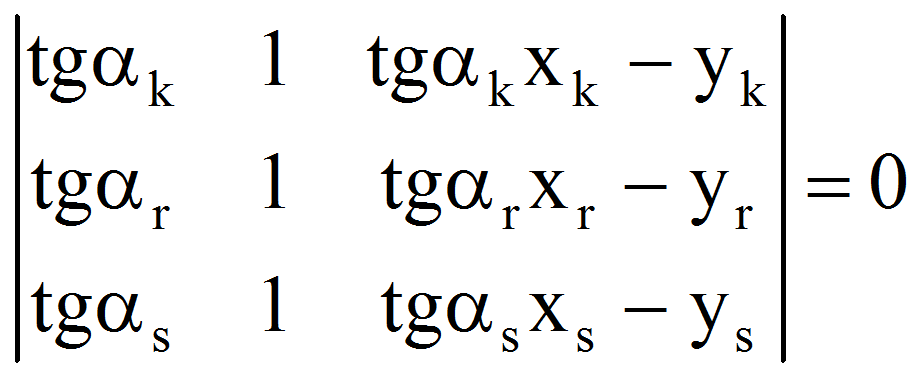(12)

equivalent to: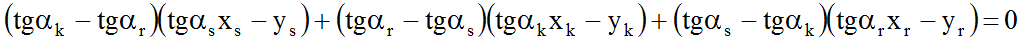(13)

the solution being given by the first two equations: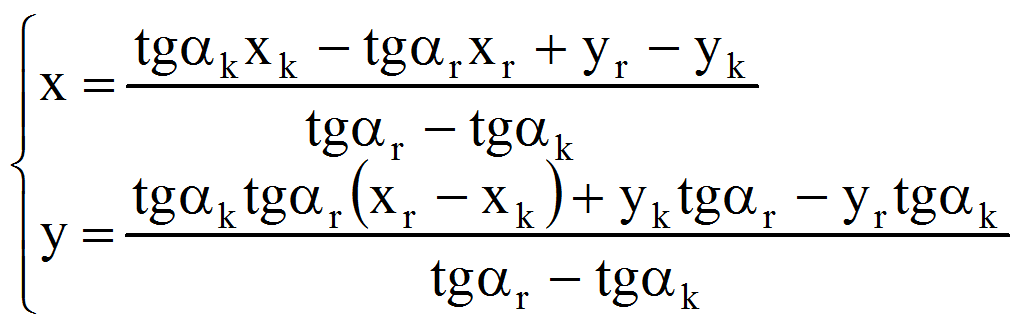(14)

Therefore, for all the solutions of the system (10) we will check the equations (13) 1krsn, those which satisfy substituting in (14) for two arbitrary values kr and determining the pairs (x, y). After that we weill replace these pairs in the characteristic system: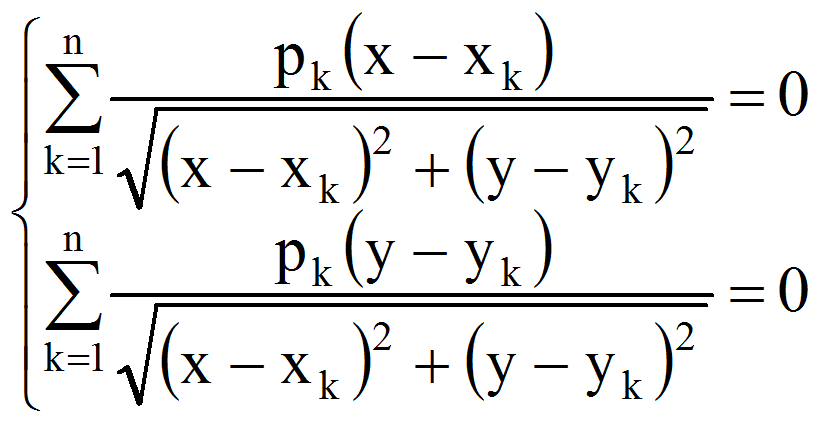(15)

If there is a pair (, ) then, finally, the minimum is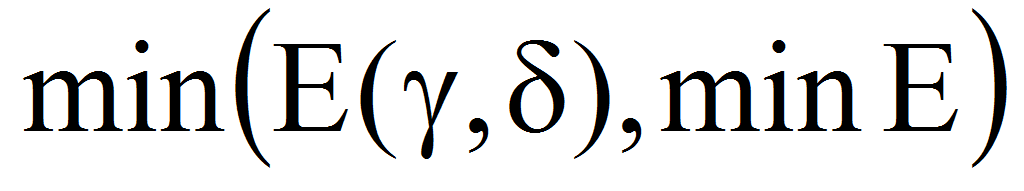. If none of the pairs (x, y) does not check the system, the minimum is minE.

4 The solution of the problem for three points

Returning to the system (10), we have for 1sn, fixed: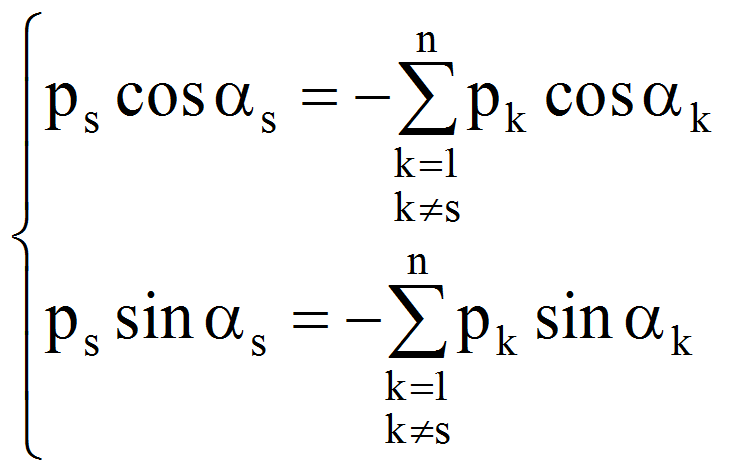(16)

Adding, after squaring, we obtain successively: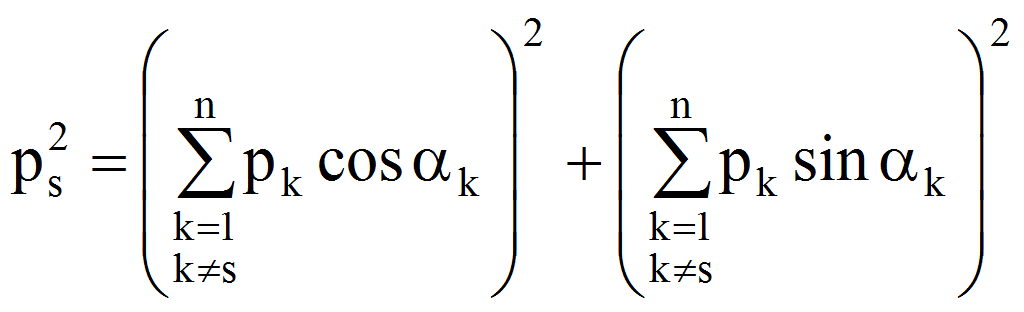(17)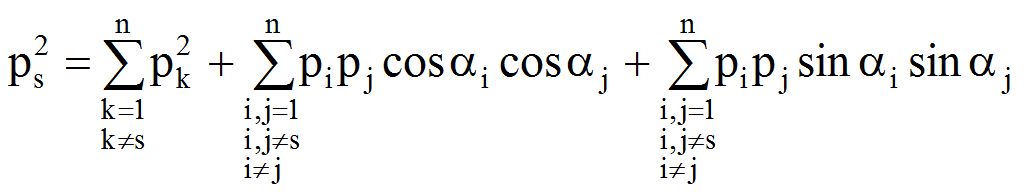(18)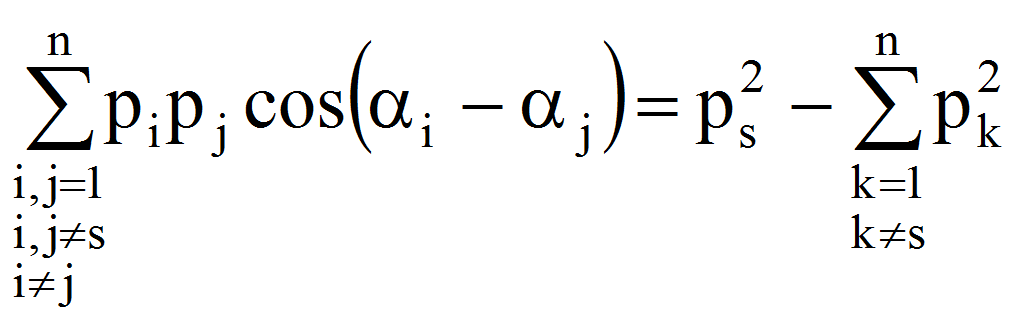, s=(19)

For n=3 we have with s=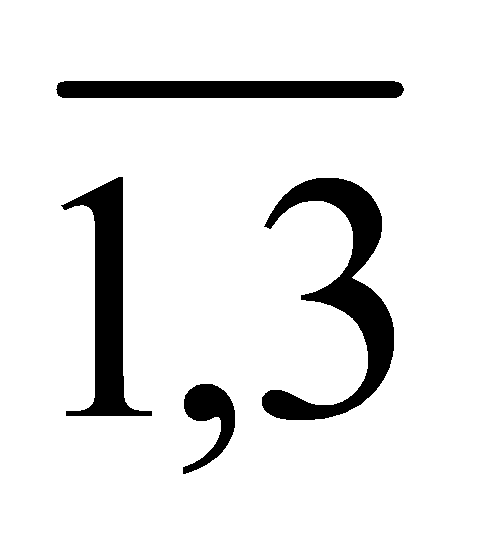: